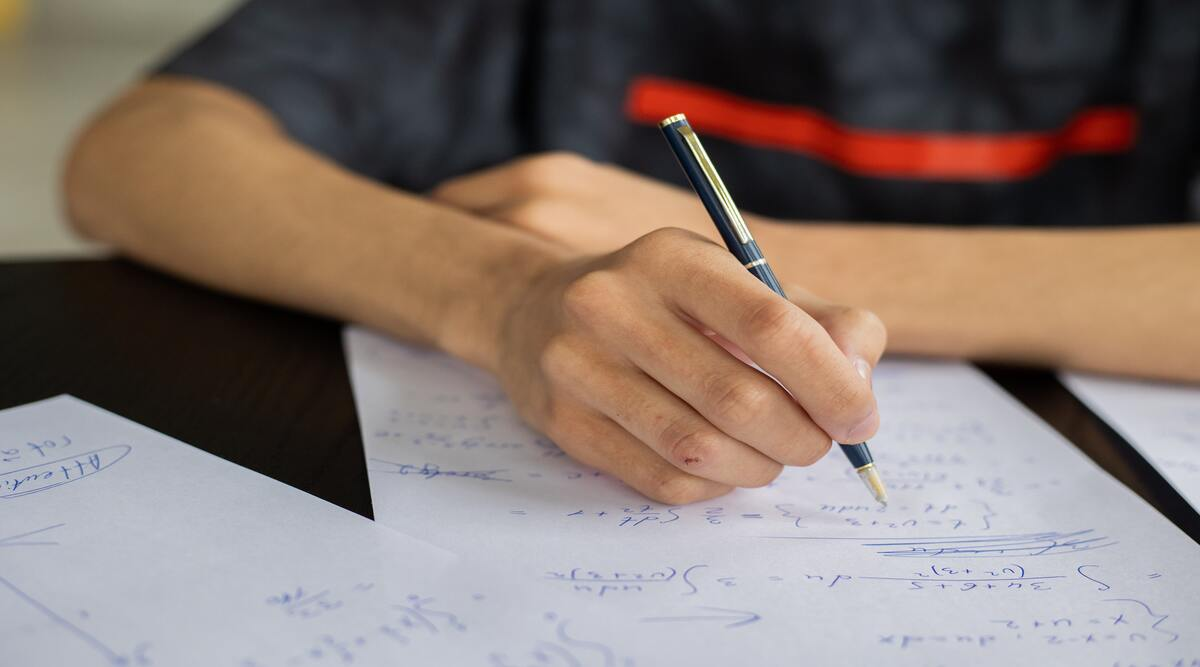Become Express Subscriber

# CBSE Class 12 Maths exam on December 6: Check tips to efficiently solve math problems in MCQ pattern

## In addition to the tips above, students should pay due attention to the time management, the accuracy of calculation, and of course, careful darkening of appropriate circles and mentioning the correct option in the specific column for answer-marking.Revising the entire course and taking a mock examination simulating the examination condition is a good idea. (Representative image)

– Sangeeta Hajela

It’s time soon to attempt the first-ever mid-term pen and paper examination to be conducted by the CBSE. The fact that it is an MCQ-based examination, makes it different from the subjective papers held thus far. Its inclusion in the final compilation of the result is a certainty, thus increasing its importance furthermore.

Appearing six months prior to the expected time for a subject like mathematics would have surely made many parents and students worried. The following tips would prove helpful for taking the examinations, especially that of Mathematics for Class 12.

To begin with, revising the entire course and taking a mock examination simulating the examination condition is a good idea. An important part of it would be learning to fill in the new OMR appropriately. Adhering to the basic instructions very effectively communicated by the board and the schools, helps one understand and approach the examinations comfortably. This is a general guideline for all subjects

Subscriber Only Stories

However, in the specific context of the subject mathematics, the following pointers can be of some help.

The traditional method of solving a problem would be to approach it step-wise, using pen and paper, and arrive at the right answer. But in an MCQ, one can adopt the elimination method, especially If the traditional method is difficult or has a long, time-consuming solution

For example: If the question is: The probability that a leap year has 53 Sundays, Answer:

(a) 2/7    (b) 3/2    (c) 0   (d) 1

Here, we know that the probability of an event cannot be more than 1 so option (b) is ruled out. We also know that probability is 1 if it is a sure event, which is not the case in the given question; so option (d) is also ruled out. The probability of an event is zero if it is an impossible event, which again is not a case.  So the only choice left is (a) which is the required correct answer.

By using shortcut methods: In mathematics, there are alternate/shortcut methods available for most of the topics, if one is aware of some of these alternate methods then the MCQ questions of the topics like trigonometry, polynomials and coordinate geometry, etc. can be solved easily and efficiently. It is a very smart and time-saving tactic.

Using figures, Graphs: The use of figures and graphs comes in handy in arriving at the right answer choice in an MCQ type of question. For example, by drawing the graphs and figures, the questions of topics like Linear Equations, Triangles Coordinate Geometry etc. can be solved easily.

Using choices to arrive at the right answer: Many a time we may arrive at the right answer just by verifying which answer choice satisfies the conditions in the given question.

For example: The solution to the linear equations 2x + y = 3 and x + 3y = 4 is

(a) (1, 2)       (b)   (2, 1)   (c)   (1, 1)     (d)     (1, -1)

Here, the only choice (c) satisfies both the equations, hence the right choice.

A Word of Caution: Extra care should be taken while applying short cut and elimination methods in solving questions that have choices like all of the above and none of the above. Questions with these kinds of choices should be solved, preferably, using the traditional method.